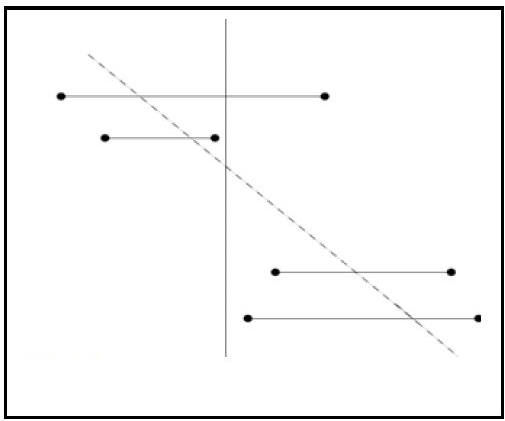# Does there exist a transversal of given set of horizontal line segments

Given a set S of N horizontal segments in the 2-D plane. Return will there exist a line which intersects all the segments in S.
A segment is represented as a pair of coordinates of its left and right end point. A line intersects a segment if it intersects the line segment defined by the two endpoints of this interval.

We have to tell about the existence, the line is not required. Expected complexity is O(n). See a example figure below. Note that the transversal may not always be vertical as shown.Are you sure expected time complexity is O(n) and not O(n\log{n})?

I searched online and found out a similar problem in David Mount’s Computational Geometry handouts, which clearly mentions O(n) is possible. Please refer to the image below.

After some searching online, I found out that such a line can be reported in O(n logn) time. But here we only need to find if such a line will exist.

If you have an algorithm to solve the problem in O(n logn) time I would love to hear that. I have solved the general problem where line segment’s need not to be horizontal in O(n^2) time. Actually this problem is from Computational Geometry Algorithms and Applications book. Please refer to the image below:

I don’t know how to do it in O(n) yet, but for an O(n\log{n}) solution, Let’s assume the line x=my+c. Let’s say the segments are denoted by l_i, the x co=ordinate of the left point, r_i, the x co-ordinate of the right point, and h_i, the y co-ordinate of the segment. For the line to intersect, a segment, l_i \le x \le r_i which implies l_i\le mh_i+c \le r_i which implies l_i-mh_i\le c \le r_i - mh_i. So we have to find m such that max(l_i-mh_i)\le c\le min(r_i-mh_i). We can therefore say that c exists if and only if max(l_i-mh_i)\le min(r_i-mh_i).
Create a new graph, which has m on the x co-ordinate.
Create two graphs. One should be the lower convex hull of l_i - mh_i, and the other should be the upper convex hull of r_i - mj_i. If there is any point where the lower convex hull is below the upper convex hull, then there exists a line.